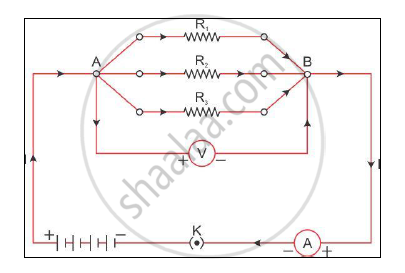# With a Neat Labelled Diagram and Derive the Equation for Three Resistances Connected in Parallel. - Science and Technology 1

With a neat labelled diagram and derive the equation for three resistances connected in parallel.

#### Solution

When two or more resistors are joined at the same end, the resistances are connected in parallel.The potential difference in parallel remains the same across all the resistors.

The current is the sum of the currents across all the individual resistors.

l = l1 + l2 + l3                                                      .........(1)

Let Rp be the resultant resistance of the circuit.

On applying Ohm’s law to the entire circuit, we get

l = V/Rp                                                          ................(2)

Now, applying Ohm’s law to individual resistances, we get

l1 = V/R1

l2 = V/R2

l3 = V/R3                                                            ................(3)

From equations (1), (2) and (3), we get

V/R_p=V/R_1+V/R_2+V/R_3

therefore1/R_p=1/R_1+1/R_2+1/R_3

Here, Rp is the resultant resistance. Thus, the reciprocal of the resultant resistance of a parallel combination of resistors is the sum of the reciprocals of individual resistances. The resultant resistance is lesser than all the resistances.

Concept: System of Resistors - Resistances in Parallel
Is there an error in this question or solution?
2013-2014 (March)

Share